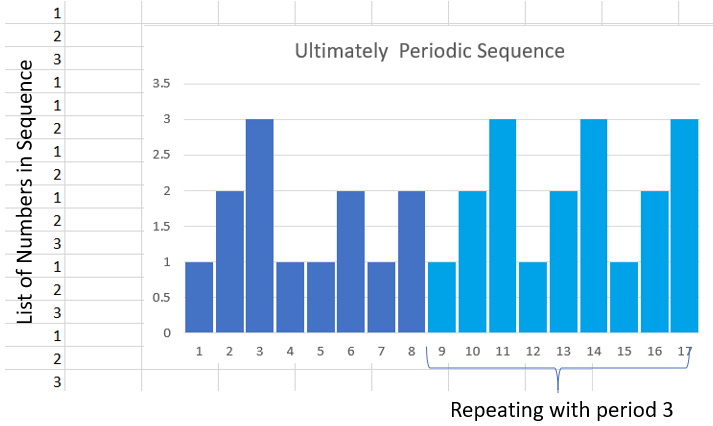# Periodic Sequence, Purely / Ultimately

Sequence and Series > Periodic sequence

## What is a Periodic Sequence?

A periodic sequence (also called a cycle or train) is a sequence that repeats itself. It is a special type of periodic function if its domain is the set of natural numbers (n = 1, 2, …).

More formally, the definition is:

ai + np = ai.

Every periodic sequence has a period, N (the length of the period or how many terms are in the repetition) so that 

x(n) = x(n + N) for all n.

As an example, the sequence {-1, 1, -1, 1,…} has period 2 and {1, 1, 1, 1, …} has period 1.

A psuedo-periodic sequence behaves in a similar way, but not quite . For example, the sequence:

{1, 2, 3, 2, 3, 4, 3, 4, 5…}

starts with 1, 2, 3. The next three terms are 1(+1), 2(+1), 3(+1), and so on: adding 1 to each string of three in the sequence.

## Examples of a Periodic Sequence

• {1, 2, 1, 2, 1, 2,…}
• The decimal expansion of 1/13 = 0.0 769230 769230…
• Periodic Binary Sequences (binary numbers that repeat): {1, 0, 1, 0, 1, 0,…) or {1, 1, 0, 1, 1, 0,…)

## Purely and Ultimately Periodic

A purely periodic sequence has all repeating terms. For example, in the sequence {1, 2, 3, 1, 2, 3, 1, 2, 3} the numbers {1, 2, 3} repeat.

If the numbers eventually get to repeat (i.e. it’s periodic from some point onwards), then the sequence is ultimately periodic (also called aperiodic or eventually periodic). For example, the ultimately periodic sequence {1, 1, 2, 1, 1, 2, 1, 2, 1, 2, 3, 1, 2, 3, 1, 2, 3, 1, 2, 3, …} starts off as non-periodic but eventually repeats {1, 2, 4} to infinity.

An example of a function that gives an eventually periodic sequence is

F = f1(n), f1(n),… fk(n),

Where fk(n) is defined as f1(n) = f(n) and fk+1(n) = f(fk(n)).

If n is a non-negative integer then the sequence is eventually periodic starting at the 4th term ( 1, 2, 7, 5, 4, 6, 5, 4, 6, 5, 4, 6) .

A great trick to finding out if a sequence is ultimately periodic is to create a bar graph from the list of terms in the sequence. A visual check may reveal a pattern.A bar graph of an ultimately periodic sequence shows that the numbers eventually repeat starting at the 9th term.

## References

 Frequency Analysis of Discrete Time Signals. Retrieved April 7, 2021 from: https://engineering.purdue.edu/~ee538/Chap4_DFTsinewaves.pdf
 MacGregor, R. Generalizing the Notion of a Periodic Sequence. Retrieved April 7, 2021 from: https://www.jstor.org/stable/2321983?seq=1
 Problem C: Eventually periodic sequence. Retrieved April 7, 2021 from: https://web.eecs.umich.edu/~kjc/acm/oct27/C.pdf

CITE THIS AS:
Stephanie Glen. "Periodic Sequence, Purely / Ultimately" From StatisticsHowTo.com: Elementary Statistics for the rest of us! https://www.statisticshowto.com/periodic-sequence-purely-ultimately/